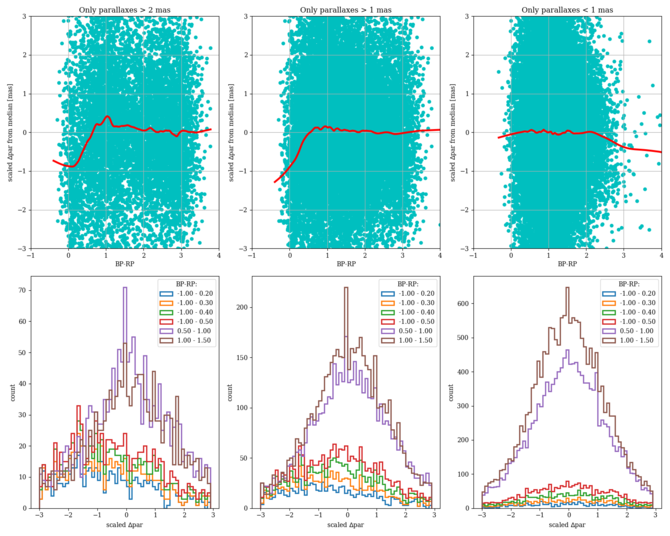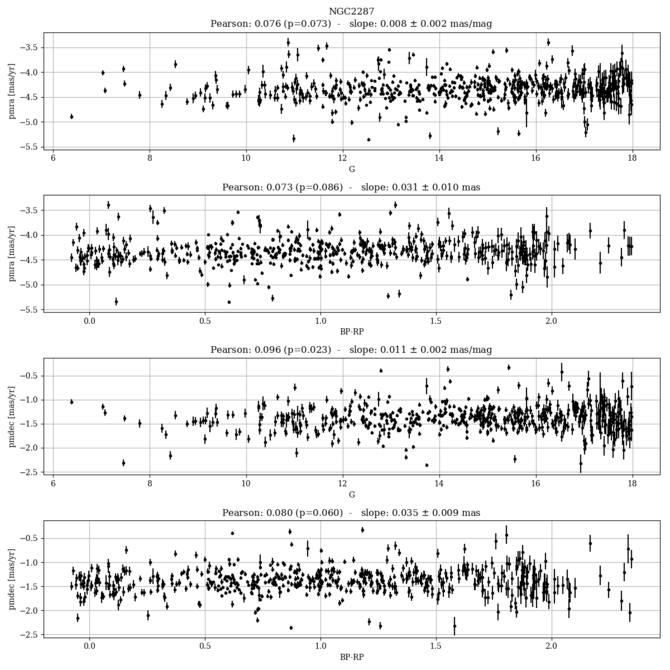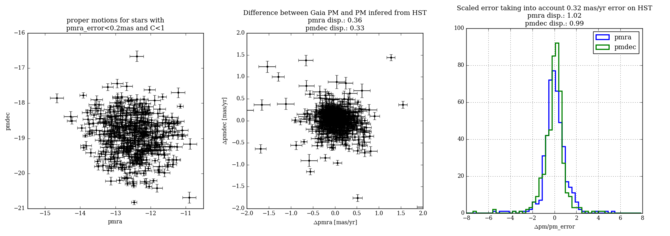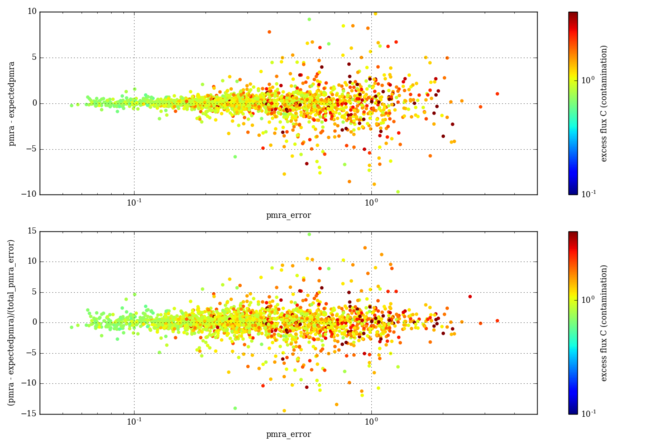# 10.7.1 Astrometry

## Parallax zero point

The analysis of about 200 clusters, including OCs and GCs, shows that there is a residual zero point. The value depends on the reference Catalogue (either MWSC or DAML), on the distance of the cluster and on the colour of the stars, and it is in the range $-0.07<(\varpi_{\rm Gaia}-\varpi_{\rm reference})<0.02$. Figure 10.37 shows the results for inflated uncertainties.Figure 10.37: Parallax differences for the whole cluster sample towards MWSC (red dots) and DAML (blue dots) reference catalogues (from Arenou et al. 2018).

## Parallax trends with magnitude and colour

We select members of clusters from their proper motions and parallaxes. In many cases, we observe a mild trends of parallax with $G$-magnitude and/or $G_{\mathrm{BP}}-G_{\mathrm{RP}}$ colour, e.g., Figure 10.38. The natural correlation between colour and magnitude along the sequence of a stellar cluster makes it difficult to tell which quantity is responsible for the trend. The small residual correlation is sometimes positive, sometimes negative. In the top right panel of Figure 10.38, the effect of the inflation of errors is seen as a discontinuity in the error distribution.

The parallax trend with colour is shown in Figure 10.39 (absolute values) and in Figure 10.40 (scaled by nominal uncertainties) for all the stars in the about 200 clusters we have analysed. We calculate the difference between the individual parallax and the median value for all the stars in the clusters. A negative trend is visible for the bluest stars of the sample ($G_{\mathrm{BP}}-G_{\mathrm{RP}}$$<0.5$), and a possible trend at the red end which might be due to the small statistics. The trend on the blue side is still significant once the the differences have been scaled to the individual nominal uncertainty (Figure 10.40), and greatly reduced for the redder stars.Figure 10.38: Top left: proper motions of stars selected as members of NGC 2423. Top middle: Gaia CMD for these stars. Top right: parallax error against G magnitude for these stars. Middle panel: parallax against G magnitude. Bottom: parallax against colour.Figure 10.39: Colour trend of the parallaxes for the members of all clusters.Figure 10.40: Colour trend with parallaxes normalised to the nominal uncertainties (from Arenou et al. 2018).

## Internal parallax dispersion

The observed parallax dispersion within a cluster should, for distant object, depend only on the uncertainties on each individual parallax measurement. We have estimated the MAD (defined as $\mathrm{MAD}(X)=1.4826\times\mathrm{median}(|X_{i}-\mathrm{median}(X)|)$) of the difference between individual parallaxes and the median of the cluster, scaled by their nominal error. The result is displayed Figure 10.41. The intrinsic parallax dispersion is evident for nearby clusters. For distant clusters, the MAD does not converge to a value of 1, as it should if the parallax uncertainties were correctly estimated, suggesting they are underestimated. This effect is even stronger in globular clusters (Figure 10.41).Figure 10.41: MAD of the distribution of the dispersion on the parallaxes scaled to the nominal uncertainties as a function of the parallaxes (in mas) for open clusters (black dots) and globulars (red crosses) (from Arenou et al. 2018).

## Proper motion trends with magnitude and colour

In fewer cases, trends in proper motions are also present (see Figure 10.42).Figure 10.42: Proper motions in right ascension and declination for NGC 2287, showing trends with G-magnitude and GBP-GRP colour.

## Mean proper motions compared with literature values

We have compared the cluster proper motions in Gaia DR2 with those listed in the catalogues of Kharchenko et al. (2013) (hereafter MWSC) and Dias et al. (2002) (hereafter DAML). A major issue with literature values of proper motions is that the two catalogues present significant differences with each other (a problem addressed in Dias et al. 2014), with no significant zero point difference but a large scatter. This obviously affects our ability to estimate the accuracy of the Gaia DR2 proper motions.

We find a residual zero point $\mu_{\rm Gaia}-\mu_{\rm reference}=0.51\pm 0.17$  mas yr${}^{-1}$, and 0.25$\pm 0.12$  mas yr${}^{-1}$ for $\mu_{\alpha}\cos\delta$ and $\mu_{\delta}$ respectively when using MWSC references. When using DAML, the differences are $0.0\pm 0.19$  mas yr${}^{-1}$, and $0.41\pm 0.18$  mas yr${}^{-1}$. These numbers are consistent with the difference between the catalogues themselves, leaving little possibility to draw strong conclusion on the proper motion zero point of the Gaia DR2 astrometry.

## Proper motion comparison with external HST data in M4

We compare the proper motions in M4 with external HST data by Bedin et al. (2013); Malavolta et al. (2015) where relative proper motions are available down to very faint magnitudes. The precision of HST proper motions would be of the order of 0.33  mas yr${}^{-1}$. When we calibrate the relation between (dx,dy) and (pmra, pmdec) using the bright stars, we find a total dispersion of about 0.35. This 0.35 is the total uncertainty due to Gaia and HST.

This field is very crowded by Gaia standards and most of the photometry is blended, which can be seen when the total $G_{\rm BP}$+$G_{\rm RP}$ flux is more than the $G$ flux. We define the colour excess $C=$ BPRPEXCESS $=2G/$($G_{\rm BP}$ +$G_{\rm RP}$). In Figure 10.43 we present the Gaia proper motions distribution, the differences in comparison with HST data, and the scaled dispersion for the stars having Gaia proper motion errors $<0.2$ mas and colour excess $C<1$. The scaled dispersion is very close to 1 both for the proper motions in right ascension and in declination, suggesting that the uncertainties are correctly estimated.

Due to the crowding in the cluster also relatively bright stars are affected by colour excess (see Figure 10.44), the scaled difference becomes larger for stars with larger Gaia uncertainties and larger colour excess. The brighter sources tend to be less affected, and sources with a larger colour excess tend to have larger astrometric uncertainties, and large scatter, but there is no strict relation. Figure 10.45 shows this effect on the right-ascension proper motion component of the proper motion (the proper motion in declination behaves in a similar way). Stars with small error bars have a proper motion in right ascension that is on average 1 error bar from the expected value. Stars with large error bars and large contamination are on average 2 or 3 error bars from the expected value, which means that either the Gaia uncertainties are severely underestimated for faint/contaminated stars.Figure 10.43: Gaia proper motion distribution in M4, the differences in comparison with HST data, and the scaled dispersion for the stars having Gaia proper motion errors <0.2 mas and colour excess C<1.Figure 10.44: The scaled dispersion of the proper motions in M4 for the stars having increasing nominal uncertainties and colour excess C.Figure 10.45: Differences of proper motions in right ascension between Gaia and HST in M4 as function of the nominal Gaia error and of the colour excess C.

We derived the weighted mean of the radial velocities in a sample of open clusters and we compare it with Dias Catalogue. Differences are very small, usually less than 1  km s${}^{-1}$. A small but significant zero point difference is present (see Figure 10.46).